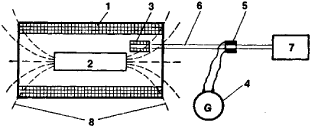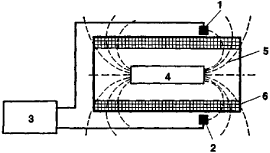# Coercimeter

Also found in: Dictionary.

## coercimeter

[‚kō‚ər′sim·əd·ər]
(engineering)
An instrument that measures the magnetic intensity of a natural magnet or electromagnet.
McGraw-Hill Dictionary of Scientific & Technical Terms, 6E, Copyright © 2003 by The McGraw-Hill Companies, Inc.
The following article is from The Great Soviet Encyclopedia (1979). It might be outdated or ideologically biased.

## Coercimeter

an instrument for measuring the coercive force of ferromagnetics. Coercimeters for measuring coercive force according to the magnetization jHc, or Hc1, are the most common, since the method of measurement is simple and, for materials with Hc < 500 amperes per meter (A/m), the values of coercive force determined by induction and magnetization differ little from each other.

To measure Hc, a test specimen is first magnetized virtually to saturation in an electromagnet or in the magnetizing coil of the coercimeter. A direct current is passed through the coil (with the specimen inside it), so that its magnetic field demagnetizes the specimen. The current is increased until the specimen’s magnetization J is reduced to zero as recorded by various types of indicators (null instruments). The demagnetizing field intensity Hc is determined from the current in the coercimeter coil that corresponds to the specimen’s condition when J = 0. For this purpose the relationship between the magnetic field intensity Hc created by the coil and the strength of the current flowing through its winding is first established. The ammeter in the circuit of the magnetizing coil often has a scale calibrated directly in units of field strength.Figure 1. Coercimeter with measuring generator (block diagram): (1) magnetizing coil, (2) specimen, (3) measuring generator coil, (4) moving-coil galvanometer connected to the brushes of commutator (5), (6) shaft of electric motor (7), (8) lines of force of specimen’s magnetic field

Coercimeters are distinguished according to the basic method of determining when the magnetization of a specimen is equal to zero.

A schematic diagram of a coercimeter apparatus with a measuring generator used as a null instrument is shown in Figure 1; equipment in which a ferromagnetic probe is the null indicator is shown in Figure 2. Ferromagnetic probes are very sensitive and can therefore be located outside the magnetizing coil, thus reducing the dependence of the instrument’s readings on the shape of the specimen.Figure 2. Coercimeter with ferromagnetic probe (block diagram): (1) and (2) sensing elements of ferromagnetic probe, connected in a differential circuit; (3) ferromagnetic-probe null instrument; (4) specimen; (5) lines of force of specimen’s magnetic field; (6) magnetizing coil

In addition to the types of coercimeter mentioned above, coercimeters with Hall transducers and with measuring coils that are connected to a ballistic galvanometer and pulled away from the specimen to determine residual magnetization—as well as vibration coercimeters, in which a vibrating measuring coil serves as an indicator—are also common.

To measure the coercive force of a specimen according to its induction (BHc), the specimen is made a part of a closed magnetic circuit of a permeameter, an electromagnet, or an “attached” coercimeter (a simplified permeameter used to determine one point of a hysteresis loop, BHc). The value of BHc corresponds to the strength of the demagnetizing field at which the induction B of the specimen is zero.

### REFERENCES

Kifer, I.I. Ispytaniia ferromagnitnykh materialov, 3rd ed. Moscow, 1969.
Magnitnye izmereniia. Edited by E. T. Chernyshev. Moscow, 1969.

I. I. KIFER